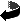# Dirac eigenvalue estimates on surfaces by Bernd Ammann, Christian Bär

Dirac eigenvalue estimates on surfaces (.pdf)
Math. Z. 240, 423-449 (2002), DOI 10.1007/s002090100392

### Abstract

We prove lower Dirac eigenvalue bounds for closed surfaces with a spin structure whose Arf invariant equals 1.
Besides the area only one geometric quantity enters in these estimates, the spin-cut-diameter $\de(M)$ which depends on the choice of spin structure. It can be expressed in terms of various distances on the surfaces or, alternatively, by stable norms of certain cohomology classes. In case of the 2-torus we obtain a positive lower bound for all Riemannian metrics and all nontrivial spin structures. For higher genus $g$ the estimate is given by $|\la| \geq {2\sqrt{\pi}\over (2g+1)\,\sqrt{\area(M)}} -{1\over \de(M)}.$
The corresponding estimate also holds for the $L^2$-spectrum of the Dirac operator on a noncompact complete surface of finite area. As a corollary we get positive lower bounds on the Willmore integral for all 2-tori embedded in $\RR^3$.

### Mathematics Subject Classification

58J50, 53C27, 53A05

### Keywords

eigenvalues of the Dirac operator, surfaces, stable norm, spin structure, Arf invariant, spin-cut-diameter, Willmore integralZurück zur Homepage

Bernd Ammann, Christian Bär,
The Paper was written on 2.4.2001
Last update 5.4.2001